Learn from home

The teachers### Vector FormIf P(x1, y1, z1) is a point on the line r and the vectorhas the same direction as, then it is equal tomultiplied by a scalar:### Parametric Form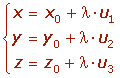### Cartesian Equations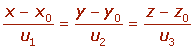A line can be determined by the intersection of two planes.### Examples

1.Find the equations of the line that pass through the point A = (1, 2, 1) and whose direction vector is.

## Parametric Form## Cartesian Equations2.Find the equations of the line that pass through the points A = (1, 0, 1) and B = (0, 1, 1).3.Given the line r: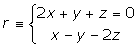Find its equations in parametric and Cartesian form.4The equation of a line r is:Do the points A = (0, −2, −2) and B = (3, 2, 6) belong to the line r?5.Find the equation of the line that is parallel to the lines given by x = 3λ, y = λ and z = 2λ + 2, and contains the point P(0, 1, −1).6.A straight line is parallel to the planes x + y = 0 and x + z = 0. If it passes through the point (2, 0, 0), find its equation.

The direction vector of the line is perpendicular to the normal vectors of each plane.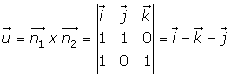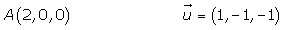Did you like the article?(2 votes, average: 5.00 out of 5)Loading...

Emma

I am passionate about travelling and currently live and work in Paris. I like to spend my time reading, gardening, running, learning languages and exploring new places.

Did you like
this resource?

Bravo!